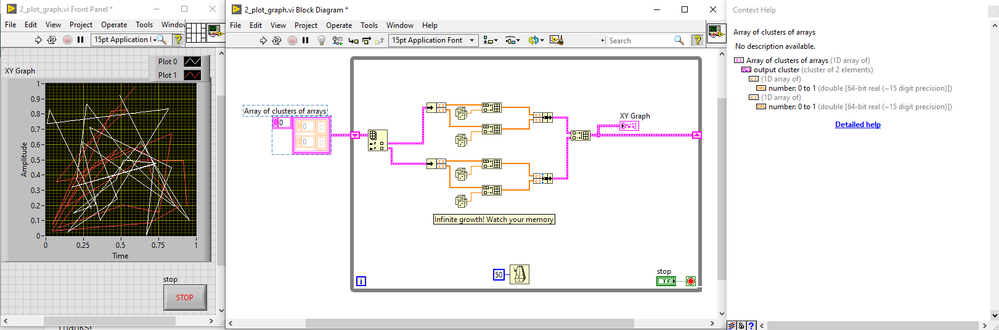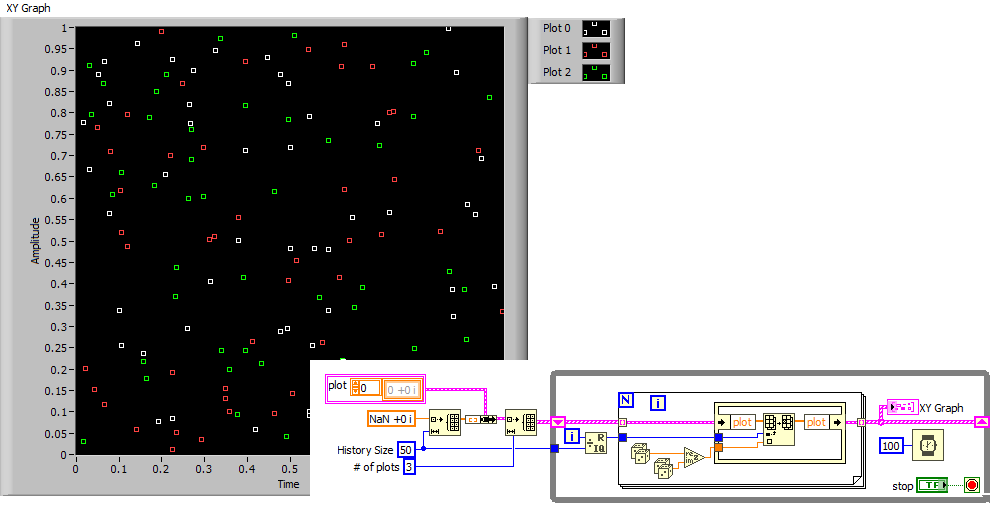# LabVIEW

cancel
Showing results for
Did you mean:

Solved!
Go to solution

## Plotting Multiple XY graphs in real time

Hi All,

I am trying to plot two sets of data in real time on a single XY graph in a while loop.  I am able to plot the two individually, but cannot get them to combine in a single chart.  When I bundle the two data points and try to input them into the graph, I get a broken wire with errors such as "type of source is 1D array of cluster of 2 elements, type of sink is 1D array of cluster of 2 elements" are these not the same?  Is there a simpler way to achieve what I am trying to do? Please see attached image of the block diagram and front panel.

Thanks!

Nick

Message 1 of 14
(1,356 Views)

## Re: Plotting Multiple XY graphs in real time

Here's an updated image of my problem - see the reason for the broken wire in the context help.

Message 2 of 14
(1,341 Views)

## Re: Plotting Multiple XY graphs in real time

chart will happily show two plots and keep it's own history. The downside is the x-scale assumes the data is always at the same interval and has only 1 global setting.

A graph can show 2 plots with different x data for every y value of a plot, but you have to manage the data yourself.

IE:Message 3 of 14
(1,316 Views)

## Re: Plotting Multiple XY graphs in real time

You didn't attach your code (contained in files with the extension ".vi", not ".png" or ".jpeg"), so we cannot see what you actually did.  You also did not describe the data you are trying to plot.

If you are plotting sampled data (taken at very regular intervals), and want to see them scroll across the screen from left to right (like a strip-chart recorder, or an oscilloscope), then you probably want a Waveform Chart, where you only need to input the Y value (as X is assumed to be "time", or "sample #", uniformly increasing).  On the other hand, if both X and Y vary as functions of time, say to trace out an input/output curve, then you might want a Graph, possibly even an XY Graph.

Since you did say "Multiple XY Graphs", here's (I hope) an explanation of how they work.  Unlike Charts, Graphs have no "memory" -- if you have 3 points plotted and you want to add a fourth point, you basically plot 4 points (three "already-plotted points" and the one "new" point).  You'll notice that the XY graph "line" is a Cluster of the X coordinates.(as an Array of how ever many points you are plotting) and Y Coordinates.  If you want to plot more than one "line", you simply make another Cluster for your other Line and create an Array of Clusters with your other plot(s).

Because a Graph must be completely redrawn whenever a new point is added, they may not be the medium of choice for rapidly-changing data.  However, given that the human eye doesn't do so well at updates faster than 20-30 frames per second, if you are only plotting a few hundred points, you probably can handle 2 plots at this frame rate, particularly if you are running the plots in parallel (see Producer/Consumer Design Pattern) with your data acquisition.

Bob Schor

Message 4 of 14
(1,283 Views)

## Re: Plotting Multiple XY graphs in real time

Hi Bob,  I attached my code here.  I am able to plot two sets of data on individual XY graphs, but combining them into a single graph doesn't seem to be as simple as creating an array of both those data sets..  How would you go about combining the two datasets in my code into a single XY graph?

Thanks,

Nick

Message 5 of 14
(1,257 Views)

## Re: Plotting Multiple XY graphs in real time

So you initialize arrays with 50 elements, then run the loop forever, meaning after 50 iterations nothing interesting will ever happen because you replace elements that don't exist. Maybe you want to cyccle through elements forever instead? (a chart has a fixed history buffer, dropping data that is too old.

The brows wires contain a 1D array of points (each point is a cluster of two scalars). XY graphs understand that.

Your pink wires contains a 1D array of clusters where each cluster elements is a cluster of two scalars (i.e. points). XY graphs don't understand that!!

Don't you want to initialize your arrays with NaN? I doubt beginning by drawing 50 points at 0,0 is logical.LabVIEW Champion.Message 6 of 14
(1,253 Views)

## Re: Plotting Multiple XY graphs in real time

Here's one possibility:LabVIEW Champion.Message 7 of 14
(1,246 Views)

## Re: Plotting Multiple XY graphs in real time

Hi Altenbach,

The 50 elements is just an arbitrary number that doesn't make sense in this example but makes sense in a much larger code I am working on that I didn't want to upload here.

I can achieve brown wires by creating an array of the two data sets, but the XY graph still wont accept them.  See the attached code.  In StevenD's example above, however, he is able to plot an xy graph using pink lines.  The context help makes this look very simple. Am I missing something?

Also thanks for the NaN tip, I didn't realize I could do that!

-NickMessage 8 of 14
(1,242 Views)

## Re: Plotting Multiple XY graphs in real time

Please consider "save for previous". I cannot open LabVIEW 2021 Vis on my current computer.LabVIEW Champion.Message 9 of 14
(1,236 Views)

## Re: Plotting Multiple XY graphs in real time

Sorry about that, I attached a 2015 version:

Message 10 of 14
(1,234 Views)